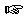### Section 3

Problem 1:
When cooking for large groups of people even small amounts of ingredients listed in a recipe can become considerable quantities. Develop a series of functions to convert between the given English liquid measuring units, then compose them to develop a function, Tsp->Barrels. Here are some useful facts: 16 Tbsp = 1 Cup; 16 Cup = 1 Gal; 42 Gal = 1 Barrel.Solution

Problem 2:
Develop a function that takes the radii of two spheres, one of which is inside the other, and computes the volume of the larger sphere not occupied by the smaller sphere. The volume of a sphere is (4 * pi * r3)/3.Solution

Problem 3:
Develop a function to calculate income tax liability. Assume that the income is over \$100,000 and is taxed according to the following schedule. To calculate income tax liability, assume that the share of an income of \$35,000 and below is federally taxed at 15%, the share of the income between \$35,000 and \$100,000 is taxed at 25%, and the remaining income over \$100,000 is taxed at 35%. Assume that there is no state income tax for income up to \$50,000, and that it is a flat 5% for all income in excess of \$50,000. Which part of the function must be modified if the state changes its tax rate?Solution

Problem 4:

Develop the function collision-speed. The function consumes the masses and velocities of two objects traveling along a track that are involved in a collision that results in the objects being stuck together. The function produces the velocity of the resulting object.

Here's some information to help you. Since the object are traveling towards each other, one of them will have a positive velocity and the other a negative one. Each object has a mass (m) and a constant velocity (v). The product of mass and velocity is called momentum. A particular collision of two objects combines the two masses and preserves the momentum. More precisely, the momentum of the resulting object is the sum of the initial two momenta. Consequently, the velocity of the new object is the result of dividing the new momentum by the combined mass.Solution

Problem 5:

Develop the function total-cost. The function consumes the selling price of a house and the amount of the down payment, then produces the grand total paid by the buyer at the end of the loan.

The following terms apply. The mortgage is financed for 30 years. The base interest rate is a simple 8.0% per year. Extra fees total \$1000.

Here are some hints: the principal is the difference between the selling price and the down payment; only the principal is used in calculating the interest; the total cost of the loan is the selling price plus the interest plus any fees.Solution

 Jamie Raymond Matthias Felleisen 01 december 2003